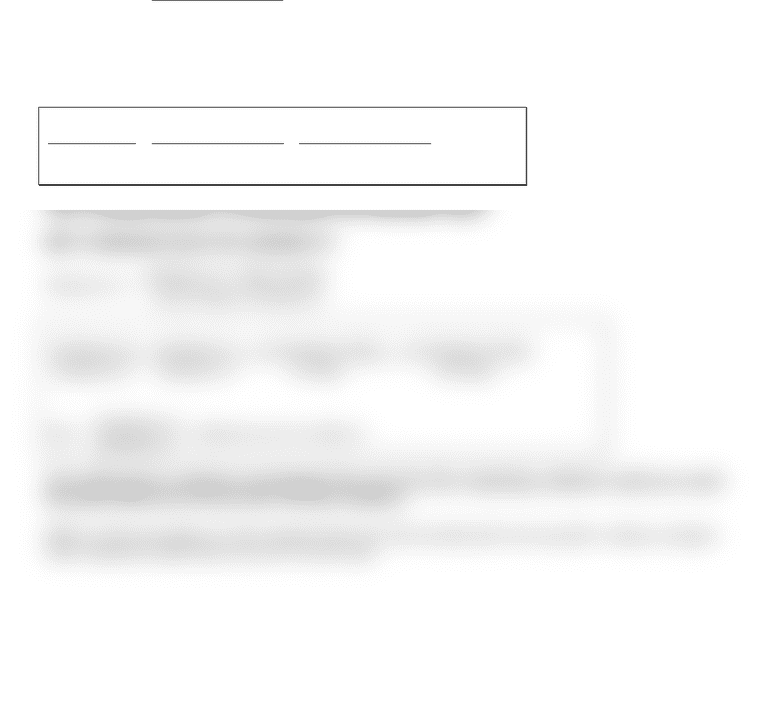Textbook Notes (290,000)
US (110,000)
UCLA (2,000)
CHEM (200)
Chapter 2

# CHEM 14BL Chapter Notes - Chapter 2: Volumetric Flask, Approximation Error

Department
Chemistry and Biochemistry
Course Code
CHEM 14BL
Professor
Henary Maher
Chapter
2

This preview shows half of the first page. to view the full 1 pages of the document.Chemistry 14BL
Calculating % Inherent Error for Experiment #2 Post-lab Report
Please read lecture guide #1 or the lab manual (p. 23 - 29) on how to calculate % relative error
for various mathematical operations BEFORE you follow the steps shown below. It will help
you to understand the formulations on this handout.
Note: [ ] means that the quantity has a unit of mol/L.
Δ
() means the absolute error of the
equipment. Refer to page 31 for absolute errors in various lab equipment.
(I) % Inherent error (or sometimes refer to as % relative error) for solution 1:
[Solution 1] = Wt. of the Food - dye
Molecular Weight
"
#
\$
%
&
' ÷(Total Volume of the Solution in Liters)
Since the only mathematical operations involved in the above equation are multiplication and
division, we can use the relative error equation that is specific design for multiplication and/or
division as outlined on lecture guide #1 or in the lab manual.
Δ [Solution 1]
[Solution 1] =Δ (Wt. of Food - dye)
(Wt. of Food - dye) +Δ (Volumetric Flask)
100.00mL
Note: we assume that there is no uncertainty in the molecular weight.
(II) % Inherent error for solution 2:
[Solution 2] = [Solution 1] * Pipet Volume
Total Volume of Solution 2
Δ [Solution 2]
[Solution 2] =Δ [Solution 1]
[Solution 1] +Δ (Volumetric Pipet)
100.00mL
Note:
Δ [Solution 1]
[Solution 1]
= inherent error for solution 1.
You will need to continue to propagate the error for the remaining solutions until you reach
the final inherent error for the very last solution.
Refer to the procedures in the manual and your lab notebook for the specific volumes of pipet
and volumetric flask that you use in each step.